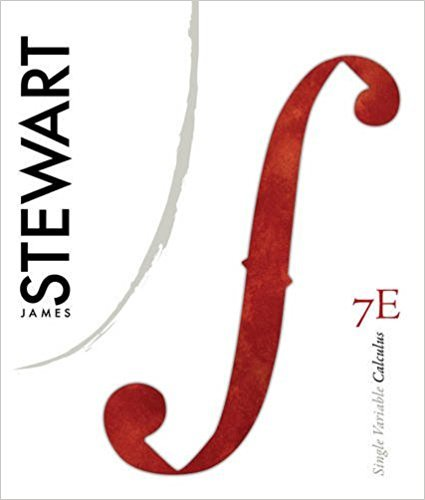×
Get Full Access to Single Variable Calculus - 7 Edition - Chapter 11 - Problem 13
Get Full Access to Single Variable Calculus - 7 Edition - Chapter 11 - Problem 13

×

# Determine whether the series is | Ch 11 - 13, 7th EditionISBN: 9780538497831 151

## Solution for problem 13 Chapter 11

Single Variable Calculus | 7th Edition

• Textbook Solutions
• 2901 Step-by-step solutions solved by professors and subject experts
• Get 24/7 help from StudySoup virtual teaching assistantsSingle Variable Calculus | 7th Edition

4 5 1 291 Reviews
31
0
Problem 13

Determine whether the series is convergent or divergent

Step-by-Step Solution:
Step 1 of 3

Step 2 of 3

Step 3 of 3

##### ISBN: 9780538497831

The answer to “Determine whether the series is convergent or divergent” is broken down into a number of easy to follow steps, and 8 words. This textbook survival guide was created for the textbook: Single Variable Calculus, edition: 7. Since the solution to 13 from 11 chapter was answered, more than 242 students have viewed the full step-by-step answer. This full solution covers the following key subjects: convergent, determine, divergent, Series, whether. This expansive textbook survival guide covers 11 chapters, and 637 solutions. Single Variable Calculus was written by and is associated to the ISBN: 9780538497831. The full step-by-step solution to problem: 13 from chapter: 11 was answered by , our top Calculus solution expert on 11/15/17, 04:21PM.

Unlock Textbook Solution